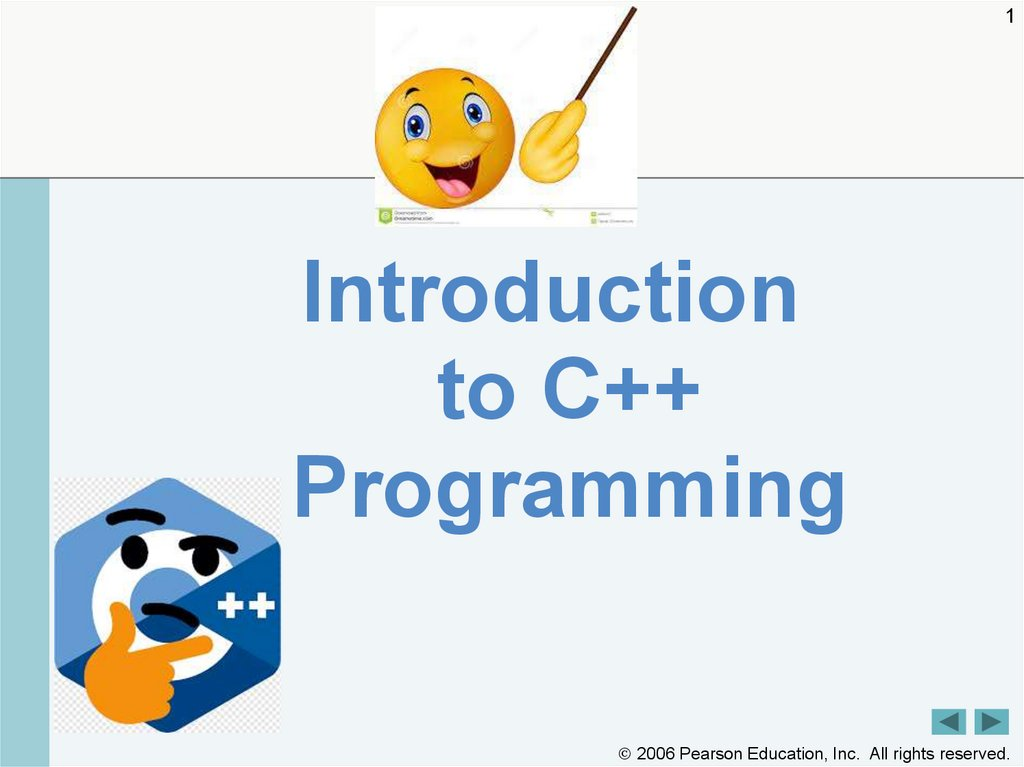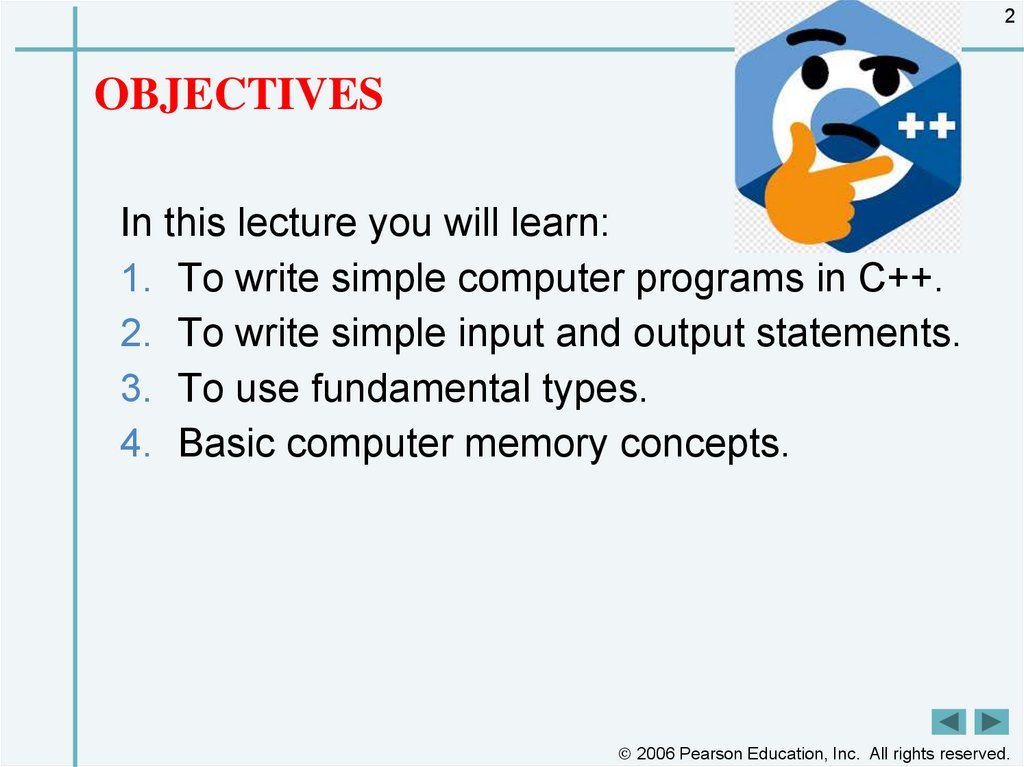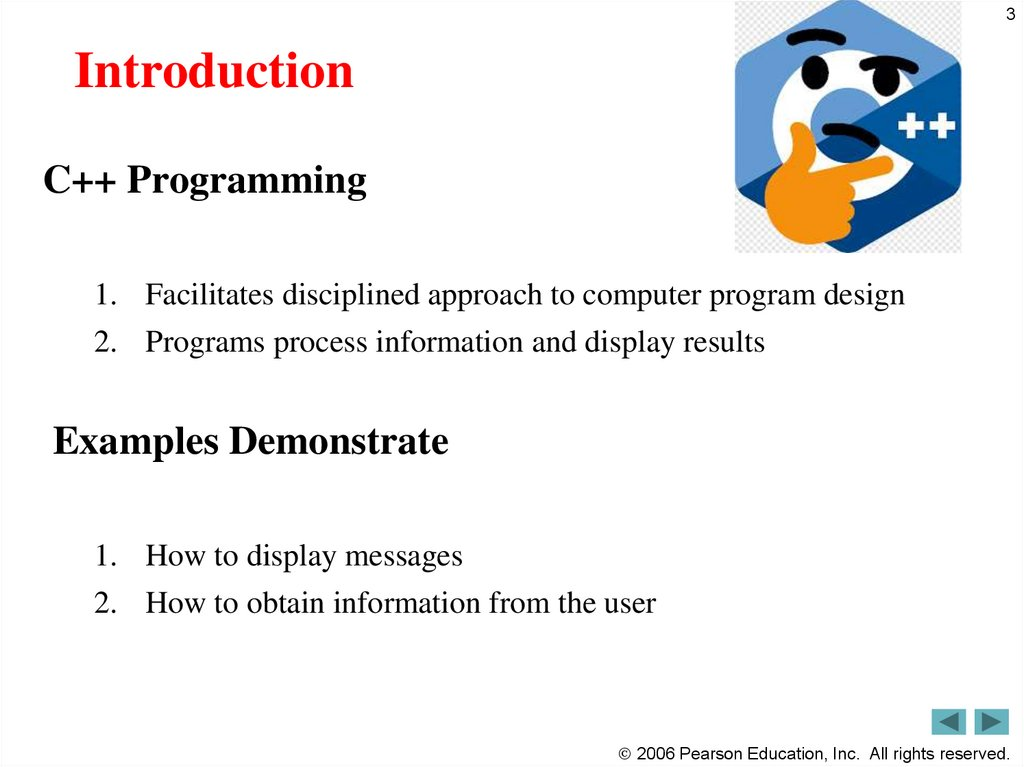# Introduction to C++. Programming

1
Introduction
to C++
Programming

## 2. OBJECTIVES

2
OBJECTIVES
In this lecture you will learn:
1. To write simple computer programs in C++.
2. To write simple input and output statements.
3. To use fundamental types.
4. Basic computer memory concepts.

## 3. Introduction

3
Introduction
C++ Programming
1. Facilitates disciplined approach to computer program design
2. Programs process information and display results
Examples Demonstrate
1. How to display messages
2. How to obtain information from the user

## 4. First Program in C++: Printing a Line of Text

4
First Program in C++: Printing a Line of Text
Simple Program
– Prints a line of text
– Illustrates several important features of C++

## 5. Outline

1
// Fig. 2.1: fig02_01.cpp
2
// Text-printing program.
3
4
5
6
7
8
#include <iostream> //
allows program
to output
to the screen
Function
main returns
an data directive
Preprocessor
to
Left
brace
{
begins
function
integer value
include input/output stream
// function main begins
execution
Statements end with a
bodyprogram
Function main
file <iostream>
int main()
exactly once in every C++ semicolon ;
{
program
std::cout << "Welcome to C++!\n"; // display message
9
10
Corresponding right brace }
ends function
Stream
insertion
Namebody
cout belongs
to operator
namespace std
main
Keyword return is one of
several means to exit a
function; value 0 indicates
that the program terminated
successfully
5
Outline
fig02_01.cpp
(1 of 1)
fig02_01.cpp
output (1 of 1)
return 0; // indicate that program ended successfully
11
12 } // end function
Welcome to C++!
2006 Pearson Education,

## 6. Good Programming Practice

6
Good Programming Practice
Every program should begin with a comment that
describes the purpose of the program, author, date and
time.

## 7. Common Programming Error

7
Common Programming Error
Forgetting to include the <iostream> header file in a
program that inputs data from the keyboard or outputs
data to the screen causes the compiler to issue an error
message, because the compiler cannot recognize
references to the stream components (e.g. cout).

## 8. Common Programming Error

8
Common Programming Error
Syntax errors are also called compiler errors, compile-time errors
or compilation errors, because the compiler detects them during the
compilation phase. You will be unable to execute your program
until you correct all the syntax errors in it. As you will see,
some compilation errors are not syntax errors.

9

10

## 11. Tokens: The smallest individual units of a program are called tokens.

11
Tokens: The smallest individual units of a program are called
tokens.
1. Constants
2. Variables
3. Keywords
4. Data Types
A C++ program is written using these tokens, white
spaces , and the syntax of the language.

## 12. Constants , Identifiers and Keywords

12
Constants , Identifiers and Keywords
The alphabets , numbers and special symbols when properly combined
form constants , identifiers and keywords.
Constant: a constant is a quantity that does not change. This can be
stored at a location in memory of computer.
Variable(identifiers) : is considered as a name given to the location
in memory where this constant is stored. Naturally the contents of
the variable can change. There are fundamental requirement of any
language. Each language has its own rules for naming these
identifiers.

## 13.

13
Following are the rules for naming identifiers:
1.
2.
3.
4.
Only alphabetic characters digits and underscores are permitted.
Uppercase and Lowercase letters are distinct
A declared keyword cannot be used as a variable name.
For Example:
3X + Y = 20

## 14. Keywords

14
Keywords
Keywords implement specific C++ language features.
They are explicitly reserved identifiers and cannot be
used as names for the program variables or other
user defined program elements.

15

## 16. Data Type

Data Type : type of data to be stored in a variable
Primitive data type (built-in data type): provided as an integral part of the
language
Integer type
Real type
int val;
2006 Pearson Education,

## 17. Primitive Data Type

Primitive Type
I
N
T
E
G
E
R
R
E
A
L
or more
or more
big
2006 Pearson Education,

## 18. Primitive Data Type

Why do we need to define the type of data?
1. Efficient use of memory space
2. Data loss can happen when store big data into small
memory space
2006 Pearson Education,

## 19. Primitive Data Type

sizeof operator
Return memory size of operand in byte
Need () when the operand is data type
Otherwise () is optional
#include <iostream>
using namespace std;
int main(void)
{
int val=10;
cout << sizeof val << endl;// print memory size
cout << sizeof(int) << endl;// print int data type
return 0;
}
2006 Pearson Education,

## 20. Primitive Data Type

Criteria of selection of data type
Real type data
Accuracy
‘double’ is common
Data type
Accuracy
6th below decimal point
15th below decimal point
More accurate than double
2006 Pearson Education,

## 21. Primitive Data Type

Example
#include <iostream>
#include <iomanip>
using namespace std;
int main(void)
{
double area;
cout << "Input radius of circle" << endl;
cout << area;
return 0;
}
2006 Pearson Education,

## 22. Primitive Data Type

unsigned: the range of data is changed
Positive integer only
Can not be used in real data type
Data type
Byte
Range
2006 Pearson Education,

## 23. Primitive Data Type

How to express letter (including characters, notations, …) inside
computer?
ASCII (American Standard Code for Information Interchange)
code was born for expressing letters.
Defined by ANSI (American National Standard Institute)
The standard of letter expression by computer
Ex) letter ‘A’ 65, letter ‘B’ 66
2006 Pearson Education,

## 24. Primitive Data Type

Range of ASCII code
0 ~ 127, possible using ‘char’ type
Declare ‘char’
Expression of letters
‘’ (quotation mark)
Change ‘A’ into 65 and store it.
Declare “store letter A into
variable ch1”
Refer ASCII code table
2006 Pearson Education,

## 25. Primitive Data Type

Example
#include <iostream>
using namespace std;
int main(void)
{
char ch1='A';
char ch2=65;
cout << ch1 <<endl << ch2 << endl;
cout << (int)ch1 << endl << (int)ch2 <<endl;
return 0;
}
2006 Pearson Education,

## 26. Primitive Data Type

ASCII code
2006 Pearson Education,

## 27. Symbolic Constant

Make ‘variable’ to ‘constant’
#include <iostream>
using namespace std;
int main(void)
{
const int MAX = 100;
const double PI = 3.1415;
return 0;
}
2006 Pearson Education,

## 28. Another C++ Program: Adding Integers

28
• Variables
– Location in memory where value can be stored
– Common data types (fundamental, primitive or built-in)
• int – integer numbers
• char – characters
• double – floating point numbers
– Declare variables with name and data type before use
• int integer1;
• int integer2;
• int sum;

## 29. Another C++ Program: Adding Integers (Cont.)

29
(Cont.)
• Variables (Cont.)
– Can declare several variables of same type in one
declaration
• Comma-separated list
• int integer1, integer2, sum;
– Variable names
• Valid identifier
– Series of characters (letters, digits, underscores)
– Cannot begin with digit
– Case sensitive (upper and lower case letter)
• Keywords

## 30. Outline

1
2
3
4
// Fig. 2.5: fig02_05.cpp
// Addition program that displays the sum of two numbers.
#include <iostream> // allows program to perform input and output
5
6
// function main begins program execution
int main()
7
8
9
{
Outline
fig02_05.cpp
Declare integer variables
// variable declarations
int number1; // first integer to add
(1 of 1)
10
11
12
int number2; // second integer to add
stream
int sum; // sum of number1 andUse
number2
13
14
15
16
17
std::cout << "Enter first
std::cin >> number1; // read first integer from user into number1
18
19
20
30
extraction
operator with standard input
integer:
prompt
user
for data
stream";to//obtain
user
input
std::cout << "Enter second integer: "; // prompt user for data
std::cin >> number2; // read second integer from user into number2
sum = number1 + number2; // add the numbers; store result in sum
21
22
std::cout << "Sum is " << sum << std::endl; // display sum; end line
23
return 0; // indicate that program ended successfully
24
25 } // end function main
Enter first integer: 45
Enter second integer: 72
Sum is 117
Stream manipulator
std::endl outputs a
newline, then “flushes output
buffer”
Concatenating, chaining or
operations
fig02_05.cpp
output (1 of 1)
2006 Pearson Education,

## 31. Good Programming Practice

31
Good Programming Practice
Place a space after each comma (,) to make

## 32. Good Programming Practice

32
Good Programming Practice
Some programmers prefer to declare each
variable on a separate line. This format allows
for easy insertion of a descriptive comment next
to each declaration.

## 33. Portability Tip

33
Portability Tip
C++ allows identifiers of any length, but your C++
implementation may impose some restrictions on
the length of identifiers. Use identifiers of 31
characters or fewer to ensure portability.

## 34. Good Programming Practice

34
Good Programming Practice
Choosing meaningful identifiers helps make a
program self-documenting—a person can
understand the program simply by reading it
rather than having to refer to manuals or

## 35. Good Programming Practice

35
Good Programming Practice
Always place a blank line between a declaration
and adjacent executable statements. This makes
the declarations stand out in the program and
contributes to program clarity.

## 36. Another C++ Program: Adding Integers (Cont.)

36
(Cont.)
• Input stream object
– std::cin from <iostream>
• Usually connected to keyboard
• Stream extraction operator >>
– Waits for user to input value, press Enter (Return) key
– Stores value in variable to right of operator
• Converts value to variable data type
• Example
– std::cin >> number1;
• Reads an integer typed at the keyboard
• Stores the integer in variable number1

## 37. Another C++ Program: Adding Integers (Cont.)

37
(Cont.)
• Assignment operator =
– Assigns value on left to variable on right
– Binary operator (two operands)
– Example:
• sum = variable1 + variable2;
– Add the values of variable1 and variable2
– Store result in sum
• Stream manipulator std::endl
– Outputs a newline
– Flushes the output buffer

## 38. Another C++ Program: Adding Integers (Cont.)

38
(Cont.)
• Concatenating stream insertion operations
– Use multiple stream insertion operators in a single statement
• Stream insertion operation knows how to output each type of data
– Also called chaining or cascading
– Example
• std::cout << "Sum is " << number1 + number2
<< std::endl;
– Outputs "Sum is “
– Then, outputs sum of number1 and number2
– Then, outputs newline and flushes output buffer

## 39. Memory Concept

39
Memory Concept
• Variable names
– Correspond to actual locations in computer's memory
• Every variable has name, type, size and value
– When new value placed into variable, overwrites old value
• Writing to memory is destructive
– Reading variables from memory nondestructive
– Example
• sum = number1 + number2;
– Value of sum is overwritten
– Values of number1 and number2 remain intact

## 40. Fig. 2.6 | Memory location showing the name and value of variable number1.

40
Fig. 2.6 | Memory location showing the name and value of variable number1.

## 41. Fig. 2.7 | Memory locations after storing values for number1 and number2.

41
Fig. 2.7 | Memory locations after storing values for number1 and number2.

## 42. Fig. 2.8 | Memory locations after calculating and storing the sum of number1 and number2.

42
Fig. 2.8 | Memory locations after calculating and storing the sum of number1 and
number2.

## 43. Arithmetic

43
Arithmetic
• Arithmetic operators
– *
• Multiplication
– /
• Division
• Integer division truncates remainder
– 7 / 5 evaluates to 1
– %
• Modulus operator returns remainder
– 7 % 5 evaluates to 2

## 44. Common Programming Error

44
Common Programming Error
Attempting to use the modulus operator (%) with
non integer operands is a compilation error.

## 45. Arithmetic (Cont.)

45
Arithmetic (Cont.)
• Straight-line form
– Required for arithmetic expressions in C++
– All constants, variables and operators appear in a straight
line
• Grouping subexpressions
– Parentheses are used in C++ expressions to group
subexpressions
• Same manner as in algebraic expressions
– Example
•a * ( b + c )
– Multiple a times the quantity b + c

## 46. Fig. 2.9 | Arithmetic operators.

46
C++ operation
C++ arithmetic
operator
Algebraic
expression
C++
expression
+
f+7
f + 7
Subtraction
-
p–c
p - c
Multiplication
*
bm or b · m
b * m
Division
/
x / y or
Modulus
%
r mod s
x
or x ÷ y
y
x / y
r % s
Fig. 2.9 | Arithmetic operators.

## 47. 2.6 Arithmetic (Cont.)

47
2.6 Arithmetic (Cont.)
• Rules of operator precedence
– Operators in parentheses evaluated first
• Nested/embedded parentheses
– Operators in innermost pair first
– Multiplication, division, modulus applied next
• Operators applied from left to right
• Operators applied from left to right

## 48. Common Programming Error 2.4

48
Common Programming Error 2.4
Some programming languages use operators **
or ^ to represent exponentiation. C++ does not
support these exponentiation operators; using
them for exponentiation results in errors.
Use pow(A, B) = A^B function in C++

## 49. Fig. 2.11 | Order in which a second-degree polynomial is evaluated.

49
Fig. 2.11 | Order in which a second-degree polynomial is evaluated.

## 50. Decision Making: Equality and Relational Operators

50
Decision Making: Equality and Relational
Operators
• Condition
– Expression can be either true or false
– Can be formed using equality or relational operators
•if statement
– If condition is true, body of the if statement executes
– If condition is false, body of the if statement does not
execute

## 51. Fig. 2.12 | Equality and relational operators.

51
Standard algebraic
C++ equality
equality or relational or relational
operator
operator
Sample
Meaning of
C++
C++ condition
condition
Relational operators
>
x > y
x is greater than y
<
x < y
x is less than y
>=
x >= y
x is greater than or equal to y
<=
x <= y
x is less than or equal to y
=
==
x == y
x is equal to y

!=
x != y
x is not equal to y
Equality operators
Fig. 2.12 | Equality and relational operators.

## 52. Common Programming Error 2.5

52
Common Programming Error 2.5
A syntax error will occur if any of the operators
==, !=, >= and <= appears with spaces between
its pair of symbols.

## 53. Common Programming Error

53
Common Programming Error
Reversing the order of the pair of symbols in any
of the operators !=, >= and <= (by writing them
as =!, => and =<, respectively) is normally a
syntax error. In some cases, writing != as =! will
not be a syntax error, but almost certainly will be
a logic error that has an effect at execution time.
(cont…)

## 54. Outline

1
2
// Fig. 2.13: fig02_13.cpp
// Comparing integers using if statements, relational operators
3
4
// and equality operators.
#include <iostream> // allows program to perform input and output
5
6
7
8
9
10
11
using std::cout; // program uses cout
using std::cin; // program uses cin
using std::endl; // program uses endl
// function main begins program
int main()
12 {
13
14
15
16
17
18
19
20
21
22
23
24
25
26
27
28
29
30
using declarations eliminate
need for std:: prefix
Declare variables
execution
54
Outline
fig02_13.cpp
(1 of 2)
int number1; // first integer
to compare
Can write
cout and
cin
without std:: prefix
statement
compares
values
cout << "Enter two integers to compare: "; //if
prompt
user for
data
If
condition is true (i.e.,
of number1
cin >> number1 >> number2; // read two integers
from user and number2 to
values are equal), execute this
test for equality
if statement comparesstatement
values
if ( number1 == number2 )
If condition
of number1 and number2
to is true (i.e.,
cout << number1 << " == " << number2 << endl;
values are not equal), execute
test for inequality
if ( number1 != number2 )
this statement
int number2; // second integer to compare
cout << number1 << " != " << number2 << endl;
if ( number1 < number2 )
cout << number1 << " < " << number2 << endl;
Compares
two numbers using
relational operator < and >
if ( number1 > number2 )
cout << number1 << " > " << number2 << endl;
2006 Pearson Education,

## 55. Outline

31
32
33
34
35
if ( number1 <= number2 )
cout << number1 << " <= " << number2 << endl;Compares
two numbers using
relational operators <= and >=
55
Outline
if ( number1 >= number2 )
cout << number1 << " >= " << number2 << endl;
36
37
return 0; // indicate that program ended successfully
38
39 } // end function main
Enter two integers to compare: 3 7
3 != 7
3 < 7
3 <= 7
fig02_13.cpp
(2 of 2)
fig02_13.cpp
output (1 of 3)
(2 of 3)
Enter two integers to compare: 22 12
22 != 12
22 > 12
22 >= 12
(3 of 3)
Enter two integers to compare: 7 7
7 == 7
7 <= 7
7 >= 7
2006 Pearson Education,